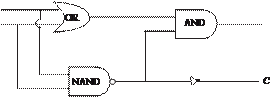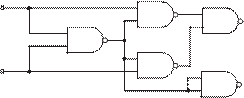### Create an Account

Home / Questions / Determine the minimum expression for the following logic function simplifying the expression

# Determine the minimum expression for the following logic function simplifying the expression

Determine the minimum expression for the following logic function, simplifying the expression:(ABC) (B· · · · · · C

#### 12.37

a.    Complete the truth table for the circuit of Figure P12.37.

12.33      A half adder circuit is shown in Figure P12.33. Write the logical expression for the outputs of this circuit in terms of its inputs. Also create a truth table for the outputs in terms of the inputs.

b.    What mathematical function does this circuit perform, and what do the outputs signify?

c.    How many standard 14-pin ICs would it take to construct this circuit?AB                                                                                          S                               xS

Jun 18 2020 View more View LessSubscribe To Get Solution